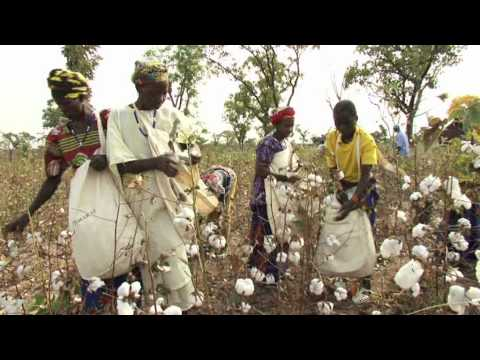•• # Risk measurement

Chapter 3 - Cotton marketing - Risk management – A cotton supply chain manager’s perspective

Since risk is an exposure to uncertain events, for measuring quantifiable risks the following steps have to be taken:

• Ascertain current exposure – captured through suitable systems.
• Measure uncertainty or volatility based on historical data – standard deviation of changes in historical prices of a commodity.

Value at Risk or VaR is the metric for measuring market risks (price, basis and currency). VaR can be defined as the maximum loss a reference set of instruments (or portfolio) can incur based on historical data and given a chosen confidence level.

For example, if VaR for a portfolio is \$1 million, then the portfolio’s loss should not exceed \$1 million with a 95% confidence level. In other words, there is a 95% probability that loss will be in the range of 0-\$1.5 million.

The steps for measuring VaR are as follows:

• Determine the portfolio holdings – for example a cotton portfolio can consist of components like West African physicals, United States physicals and cotton futures across various terminal months in CSCE (Coffee, Sugar and Cocoa Exchange).
• Calculate the standard deviation for each component
• Calculate the correlation of each component with respect to all other components. For example if there are three components C1, C2 and C3 in a portfolio, then correlation has to be calculated for C1 & C2, C1 & C3 and C2 & C3.• Calculate VaR by applying the general result.

In the above example, σ is the standard deviation of the portfolio in dollar terms, si is the component holding in quantity terms, σi is the standard deviation of component in dollars per ton terms, and ρij is the correlation factor between two components.

Multiply the portfolio ρ by the requisite confidence level (CL) to calculate VaR. For example, to calculate VaR for 95% CL, multiply σ by 1.645 and for 99% CL multiply by 2.33.

The VaR numbers and quantity exposure should be compared with the risk limit (both VaR and quantity) for ongoing risk control.•• Region:
Country:
Type:
Date from:
Date to:
••••